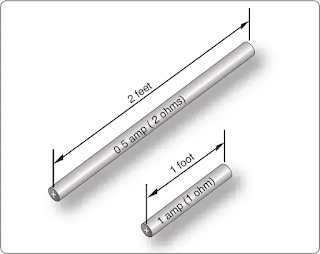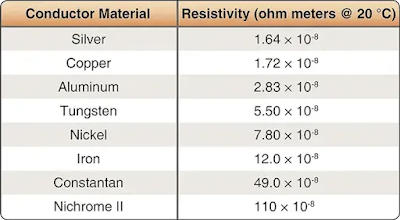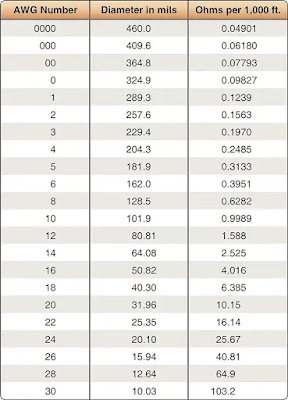# Resistance of a Conductor

While wire of any size or resistance value may be used, the word “conductor” usually refers to materials that offer low resistance to current flow, and the word “insulator” describes materials that offer high resistance to current. There is no distinct dividing line between conductors and insulators; under the proper conditions, all types of material conduct some current. Materials offering a resistance to current flow midway between the best conductors and the poorest conductors (insulators) are sometimes referred to as “semiconductors,” and find their greatest application in the field of transistors.

The best conductors are materials, chiefly metals, which possess a large number of free electrons; conversely, insulators are materials having few free electrons. The best conductors are silver, copper, gold, and aluminum; but some nonmetals, such as carbon and water, can be used as conductors. Materials such as rubber, glass, ceramics, and plastics are such poor conductors that they are usually used as insulators. The current flow in some of these materials is so low that it is usually considered zero. The unit used to measure resistance is called the ohm. The symbol for the ohm is the Greek letter omega (Ω). In mathematical formulas, the capital letter “R” refers to resistance. The resistance of a conductor and the voltage applied to it determine the number of amperes of current flowing through the conductor. Thus, 1 ohm of resistance limits the current flow to 1 ampere in a conductor to which a voltage of 1 volt is applied.

## Factors Affecting Resistance

• The resistance of a metallic conductor is dependent on the type of conductor material. It has been pointed out that certain metals are commonly used as conductors because of the large number of free electrons in their outer orbits. Copper is usually considered the best available conductor material, since a copper wire of a particular diameter offers a lower resistance to current flow than an aluminum wire of the same diameter. However, aluminum is much lighter than copper, and for this reason, as well as cost considerations, aluminum is often used when the weight factor is important.
• The resistance of a metallic conductor is directly proportional to its length. The longer the length of a given size of wire, the greater the resistance. Figure 1 shows two wire conductors of different lengths. If 1 volt of electrical pressure is applied across the two ends of the conductor that is 1 foot in length, and the resistance to the movement of free electrons is assumed to be 1 ohm, the current flow is limited to 1 ampere. If the same size conductor is doubled in length, the same electrons set in motion by the 1 volt applied now find twice the resistance; consequently, the current flow is reduced by one-half.Figure 1. Resistance varies with length of conductor

• The resistance of a metallic conductor is inversely proportional to the cross-sectional area. This area may be triangular or even square, but is usually circular. If the cross-sectional area of a conductor is doubled, the resistance to current flow is reduced in half. This is true because of the increased area in which an electron can move without collision or capture by an atom. Thus, the resistance varies inversely with the cross-sectional area of a conductor.
• The fourth major factor influencing the resistance of a conductor is temperature. Although some substances, such as carbon, show a decrease in resistance as the ambient (surrounding) temperature increases, most materials used as conductors increase in resistance as temperature increases. The resistance of a few alloys, such as constantan and Manganin™, change very little as the temperature changes. The amount of increase in the resistance of a 1 ohm sample of a conductor, per degree rise in temperature above 0° Centigrade (C), the assumed standard, is called the temperature coefficient of resistance. For each metal, this is a different value. For example, for copper the value is approximately 0.00427 ohm. Thus, a copper wire having a resistance of 50 ohms at a temperature of 0 °C has an increase in resistance of 50 × 0.00427, or 0.214 ohm, for each degree rise in temperature above 0 °C. The temperature coefficient of resistance must be considered where there is an appreciable change in temperature of a conductor during operation. Charts listing the temperature coefficient of resistance for different materials are available. Figure 2 shows a table for “resistivity” of some common electric conductors.Figure 2. Resistivity table

The resistance of a material is determined by four properties: material, length, area, and temperature. The first three properties are related by the following equation at T = 20 °C (room temperature):

Where R = resistance in ohms
ρ = resistivity of the material in circular mil-ohms per foot
l = length of the sample in feet
A = area in circular mils

## Resistance and Relation to Wire Sizing

### Circular Conductors (Wires/Cables)

Because it is known that the resistance of a conductor is directly proportional to its length, and if we are given the resistance of the unit length of wire, we can readily calculate the resistance of any length of wire of that particular material having the same diameter. Also, because it is known that the resistance of a conductor is inversely proportional to its cross-sectional area, and if we are given the resistance of a length of wire with unit cross-sectional area, we can calculate the resistance of a similar length of wire of the same material with any cross-sectional area. Therefore, if we know the resistance of a given conductor, we can calculate the resistance for any conductor of the same material at the same temperature. From the relationship:

It can also be written:

If we have a conductor that is 1 meter (m) long with a cross-sectional area of 1 (millimeter) mm2 and has a resistance of 0.017 ohm, what is the resistance of 50 m of wire from the same material but with a cross-sectional area of 0.25 mm2?

While the SI units are commonly used in the analysis of electric circuits, electrical conductors in North America are still being manufactured using the foot as the unit length and the mil (one thousandth of an inch) as the unit of diameter. Before using the equation R = × l) to calculate the resistance of a conductor of a given American wire gauge (AWG) size, the cross-sectional area in square meters must be determined using the conversion factor 1 mil = 0.0254 mm. The most convenient unit of wire length is the foot. Using these standards, the unit of size is the mil-foot. Thus, a wire has unit size if it has a diameter of 1 mil and length of 1 foot.

In the case of using copper conductors, we are spared the task of tedious calculations by using a table as shown in Figure 3. Note that cross-sectional dimensions listed on the table are such that each decrease of one gauge number equals a 25 percent increase in the cross-sectional area. Because of this, a decrease of three gauge numbers represents an increase in cross-sectional area of approximately 2:1. Likewise, change of ten wire gauge numbers represents a 10:1 change in cross-sectional area—also, by doubling the cross-sectional area of the conductor, the resistance is cut in half. A decrease of three wire gauge numbers cuts the resistance of the conductor of a given length in half.Figure 3. Conversion table when using copper conductors

## Rectangular Conductors (Bus Bars)

To compute the cross-sectional area of a conductor in square mils, the length in mils of one side is squared. In the case of a rectangular conductor, the length of one side is multiplied by the length of the other. For example, a common rectangular bus bar (large, special conductor) is 3⁄8 inch thick and 4 inches wide. The 3⁄8-inch thickness may be expressed as 0.375 inch. Since 1,000 mils equal 1 inch, the width in inches can be converted to 4,000 mils. The cross-sectional area of the rectangular conductor is found by converting 0.375 to mils (375 mils × 4,000 mils = 1,500,000 square mils).

RELATED POSTS